# The HOMFLY Braidor Algebra

(diff) ← Older revision | Latest revision (diff) | Newer revision → (diff)

## The Algebra

Let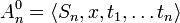$A^0_n=\langle S_n, x, t_1,\ldots t_n\rangle$ be the free associative (but non-commutative) algebra generated by the elements of the symmetric group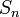$S_n$ on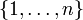$\{1,\ldots,n\}$ and by formal variables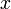$x$ and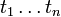$t_1\ldots t_n$, and let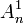$A^1_n$ be the quotient of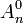$A^0_n$ by the following "HOMFLY" relations:

1.$x$ commutes with everything else.
2. The product of permutations is as in the symmetric group$S_n$.
3. If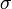$\sigma$ is a permutation then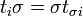$t_i\sigma=\sigma t_{\sigma i}$.
4.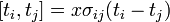$[t_i,t_j]=x\sigma_{ij}(t_i-t_j)$, where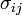$\sigma_{ij}$ is the transposition of$i$ and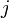$j$.

Finally, declare that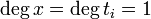$\deg x=\deg t_i=1$ while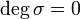$\deg\sigma=0$ for every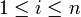$1\leq i\leq n$ and every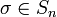$\sigma\in S_n$, and let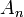$A_n$ be the graded completion of$A^1_n$.

We say that an element of$A_n$ is "sorted" if it is written in the form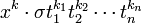$x^k\cdot\sigma t_1^{k_1}t_2^{k_2}\cdots t_n^{k_n}$ where$\sigma$ is a permutation and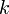$k$ and the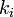$k_i$'s are all non-negative integer. The HOMFLY relations imply that every element of$A_n$ is a linear combinations of sorted elements. Thus as a vector space,$A_n$ can be identified with the ring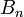$B_n$ of power series in the variables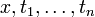$x,t_1,\ldots,t_n$ tensored with the group ring of$S_n$. The product of$A_n$ is of course very different than that of$B_n$.

Examples.

1. The general element of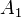$A_1$ is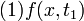$(1)f(x,t_1)$ where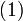$(1)$ denotes the identity permutation and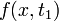$f(x,t_1)$ is a power series in two variables$x$ and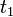$t_1$.$A_1$ is commutative.
2. The general element of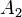$A_2$ is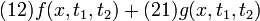$(12)f(x,t_1,t_2)+(21)g(x,t_1,t_2)$ where$f$ and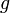$g$ are power series in three variables and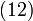$(12)$ and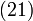$(21)$ are the two elements of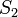$S_2$.$A_2$ is not commutative and its product is non-trivial to describe.
3. The general element of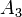$A_3$ is described using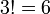$3!=6$ power series in 4 variables. The general element of$A_n$ is described using n! power series in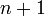$n+1$ variables.

The algebra$A_n$ embeds in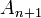$A_{n+1}$ in a trivial way by regarding$\{1,\ldots,n\}$ as a subset of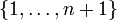$\{1,\ldots,n+1\}$ in the obvious manner; thus when given an element of$A_n$ we are free to think of it also as an element of$A_{n+1}$. There is also a non-trivial map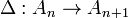$\Delta:A_n\to A_{n+1}$ defined as follows:

1.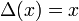$\Delta(x)=x$.
2.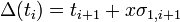$\Delta(t_i)=t_{i+1}+x\sigma_{1,i+1}$.
3.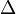$\Delta$ acts on permutations by "shifting them one unit to the right", i.e., by identifying$\{1,\ldots,n\}$ with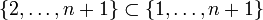$\{2,\ldots,n+1\}\subset\{1,\ldots,n+1\}$.

## The Equations

We seek to find a "braidor"; an element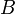$B$ of$A_2$ satisfying:

•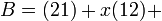$B=(21)+x(12)+$(higher order terms).
•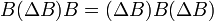$B(\Delta B)B=(\Delta B)B(\Delta B)$ in$A_3$.

With the vector space identification of$A_n$ with$B_n$ in mind, we are seeking two power series of three variables each, whose low order behaviour is specified and which are required to satisfy 6 functional equations written in terms of 4 variables.

## A Solution

The first few terms of a solution can be computed using a computer, as shown below. But a true solution, written in a functional form, is still missing.

## Computer Games

A primitive mathematica program to play with these objects is here.

## A Numerology Problem

Question. Can you find nice formulas for the functions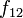$f_{12}$ and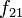$f_{21}$ of the variables$t_1$,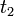$t_2$ and$x$, whose Taylor expansions begin with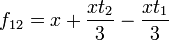$f_{12}=x+\frac{x t_2}{3}-\frac{x t_1}{3}$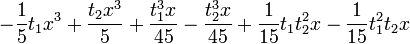$-\frac{1}{5} t_1 x^3+\frac{t_2 x^3}{5}+\frac{t_1^3 x}{45}-\frac{t_2^3 x}{45}+\frac{1}{15} t_1 t_2^2 x-\frac{1}{15} t_1^2 t_2 x$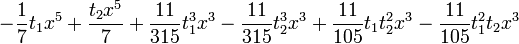$-\frac{1}{7} t_1 x^5+\frac{t_2 x^5}{7}+\frac{11}{315} t_1^3 x^3-\frac{11}{315} t_2^3 x^3+\frac{11}{105} t_1 t_2^2 x^3-\frac{11}{105} t_1^2 t_2 x^3$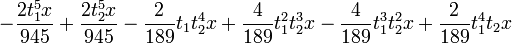$-\frac{2 t_1^5 x}{945}+\frac{2 t_2^5 x}{945}-\frac{2}{189} t_1 t_2^4 x+\frac{4}{189} t_1^2 t_2^3 x-\frac{4}{189} t_1^3 t_2^2 x+\frac{2}{189} t_1^4 t_2 x$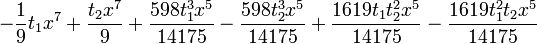$-\frac{1}{9} t_1 x^7+\frac{t_2 x^7}{9}+\frac{598 t_1^3 x^5}{14175}-\frac{598 t_2^3 x^5}{14175}+\frac{1619 t_1 t_2^2 x^5}{14175}-\frac{1619 t_1^2 t_2 x^5}{14175}$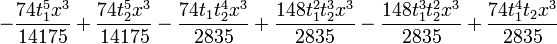$-\frac{74 t_1^5 x^3}{14175}+\frac{74 t_2^5 x^3}{14175}-\frac{74 t_1 t_2^4 x^3}{2835}+\frac{148 t_1^2 t_2^3 x^3}{2835}-\frac{148 t_1^3 t_2^2 x^3}{2835}+\frac{74 t_1^4 t_2 x^3}{2835}$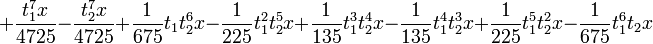$+\frac{t_1^7 x}{4725}-\frac{t_2^7 x}{4725}+\frac{1}{675} t_1 t_2^6 x-\frac{1}{225} t_1^2 t_2^5 x+\frac{1}{135} t_1^3 t_2^4 x-\frac{1}{135} t_1^4 t_2^3 x+\frac{1}{225} t_1^5 t_2^2 x-\frac{1}{675} t_1^6 t_2 x$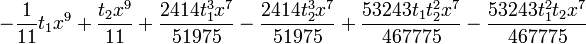$-\frac{1}{11} t_1 x^9+\frac{t_2 x^9}{11}+\frac{2414 t_1^3 x^7}{51975}-\frac{2414 t_2^3 x^7}{51975}+\frac{53243 t_1 t_2^2 x^7}{467775}-\frac{53243 t_1^2 t_2 x^7}{467775}$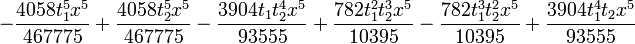$-\frac{4058 t_1^5 x^5}{467775}+\frac{4058 t_2^5 x^5}{467775}-\frac{3904 t_1 t_2^4 x^5}{93555}+\frac{782 t_1^2 t_2^3 x^5}{10395}-\frac{782 t_1^3 t_2^2 x^5}{10395}+\frac{3904 t_1^4 t_2 x^5}{93555}$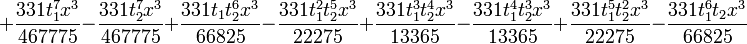$+\frac{331 t_1^7 x^3}{467775}-\frac{331 t_2^7 x^3}{467775}+\frac{331 t_1 t_2^6 x^3}{66825}-\frac{331 t_1^2 t_2^5 x^3}{22275}+\frac{331 t_1^3 t_2^4 x^3}{13365}-\frac{331 t_1^4 t_2^3 x^3}{13365}+\frac{331 t_1^5 t_2^2 x^3}{22275}-\frac{331 t_1^6 t_2 x^3}{66825}$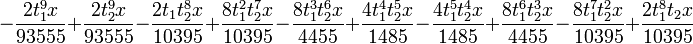$-\frac{2 t_1^9 x}{93555}+\frac{2 t_2^9 x}{93555}-\frac{2 t_1 t_2^8 x}{10395}+\frac{8 t_1^2 t_2^7 x}{10395}-\frac{8 t_1^3 t_2^6 x}{4455}+\frac{4 t_1^4 t_2^5 x}{1485}-\frac{4 t_1^5 t_2^4 x}{1485}+\frac{8 t_1^6 t_2^3 x}{4455}-\frac{8 t_1^7 t_2^2 x}{10395}+\frac{2 t_1^8 t_2 x}{10395}$

and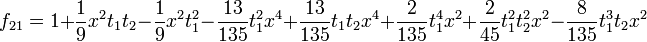$f_{21}=1+\frac{1}{9} x^2 t_1 t_2-\frac{1}{9} x^2 t_1^2 -\frac{13}{135} t_1^2 x^4+\frac{13}{135} t_1 t_2 x^4+\frac{2}{135} t_1^4 x^2+\frac{2}{45} t_1^2 t_2^2 x^2-\frac{8}{135} t_1^3 t_2 x^2$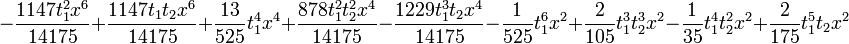$-\frac{1147 t_1^2 x^6}{14175}+\frac{1147 t_1 t_2 x^6}{14175}+\frac{13}{525} t_1^4 x^4+\frac{878 t_1^2 t_2^2 x^4}{14175}-\frac{1229 t_1^3 t_2 x^4}{14175}-\frac{1}{525} t_1^6 x^2+\frac{2}{105} t_1^3 t_2^3 x^2-\frac{1}{35} t_1^4 t_2^2 x^2+\frac{2}{175} t_1^5 t_2 x^2$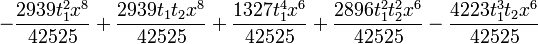$-\frac{2939 t_1^2 x^8}{42525}+\frac{2939 t_1 t_2 x^8}{42525}+\frac{1327 t_1^4 x^6}{42525}+\frac{2896 t_1^2 t_2^2 x^6}{42525}-\frac{4223 t_1^3 t_2 x^6}{42525}$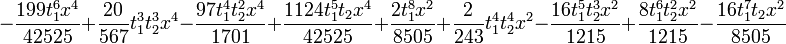$-\frac{199 t_1^6 x^4}{42525}+\frac{20}{567} t_1^3 t_2^3 x^4-\frac{97 t_1^4 t_2^2 x^4}{1701}+\frac{1124 t_1^5 t_2 x^4}{42525}+\frac{2 t_1^8 x^2}{8505}+\frac{2}{243} t_1^4 t_2^4 x^2-\frac{16 t_1^5 t_2^3 x^2}{1215}+\frac{8 t_1^6 t_2^2 x^2}{1215}-\frac{16 t_1^7 t_2 x^2}{8505}$?